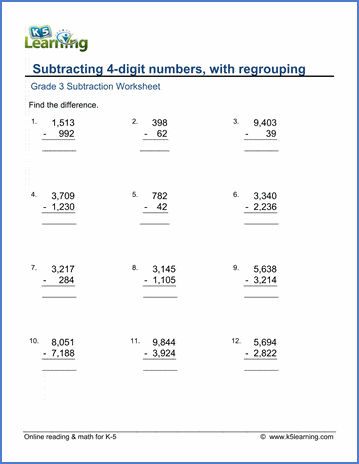# Subtracting 4-digit numbers in columns

## Worksheets: Subtracting 4-digit numbers in columns, with regrouping

Below are six versions of our grade 3 math worksheet on subtracting random numbers from numbers up to 9,999. The problems include the need for regrouping (or "borrowing") and are shown in columnar form. These worksheets are pdf files.## More subtraction worksheets

Explore all of our subtraction worksheets, from subtracting by counting objects to subtracting large numbers in columns.

## What is K5?

K5 Learning offers reading and math worksheets, workbooks and an online reading and math program for kids in kindergarten to grade 5.  We help your children build good study habits and excel in school.